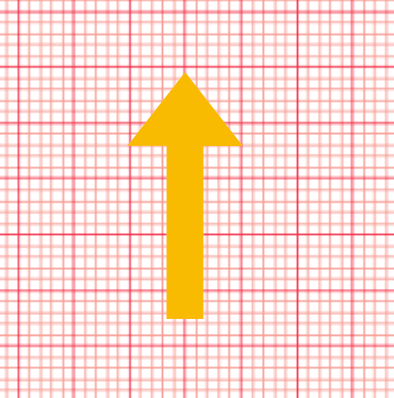Like neuroanatomy, the ECG is a classic thing to fill medical students with dread each year. Take it one step at a time, particularly in the preclinical years, and get these core concepts of rate, rhythm and axis right – along with some core electrochemistry – to hold you in good stead in the later years as you correlate pathology. Good luck, and take is slow!

Reviewed by: awaiting review

## Introduction to the ECG

Congratulations - you have completed Introduction to the ECG. You scored %%SCORE%% out of %%TOTAL%%. Your performance has been rated as %%RATING%%
 Question 1
Which of the following is not true of the ECG trace?
 A Sinus rhythm is given as a P wave evenly placed before each QRS complex B The PR interval is from the start of the P wave to the end of the R wave C ST is an isoelectric trace where the ventricles are in complete depolarisation D The QT interval is the time it takes for complete ventricular depolarisation and repolarisation
 Question 2
The small positive deflection seen post-QRS complex is indicative of
 A Positive charge to positive electrode B Negative charge to negative electrode C Positive charge to negative electrode D Negative charge to negative electrode
Question 2 Explanation:
A fundamental construct of electrophysiology is that a negative current flowing toward a negative electrode will elicit a positive deflection. In the repolarsation of the membrane, such occurs.
 Question 3
In a 12-lead ECG, where is V5 placed?
 A Fourth intercostal space, parasternally right side B Site of the apex beat of the heart C Left anterior axillary line D Left mid-axillary line E Left posterior axillary line
 Question 4
The right ventricular electrical amplitude is best represented in which of the following leads?
 A V4 B V5 C V6 D V7
 Question 5
Using convention, which limb electrode is "neutral"?
 A Left arm B Left leg C Right arm D Right leg
 Question 6
Which electrode would you use to visualise the electrical activity in the region of the heart supplied by the circumflex branch of the left coronary artery?
 A V1 B II C V3 D aVL
Question 6 Explanation:
There are four electrodes used for this territory (which is the left, lateral aspect of the heart): V5, V6, I, aVL
 Question 7
The time between the two ventricular depolarisation complexes is termed?
 A PR interval B RR interval C QRS complex D QT interval
 Question 8
How many electrodes are attached to the patient in a 12-lead ECG?
 A 10 B 11 C 12 D 13
Question 8 Explanation:
A "lead" is a point between two electrodes. As one electrode is the ground, we have ten electrodes to utilise.
 Question 9
How much time has elapsed across seven small squares of an ECG trace?
 A 0.30 seconds B 0.28 seconds C 1 second D 0.5 seconds
 Question 10
 A aVR is negative, aVL is positive B aVR is negative aVF is positive C aVL is negative, aVF is positive D aVR is negative aVF is positive
 Question 11
The axis represented in the image of this question is indicative of which modality?A Charge B Amplitude C Voltage D Time
Question 11 Explanation:
On an ECG paper trace, the horizontal axis is time, the vertical is voltage.
 Question 12
A patient has 6 big squares between their RR interval. Calculate the patient's ECG rate.
 A 50 B 55 C 60 D 65
Question 12 Explanation:
ECG rate = 300/big squares = 300/6 = 50
 Question 13
The first step in ascertaining a QRS axis is
 A To find the equiphasic lead B To move 90º to the isoelectric line C To count rate D To count rhythm
 Question 14
 A -30º and +90º B -30º and 0º C 0º and +90º D +90º and +180º
 Question 15
Which of the following would not be responsible for a change of cardiac axis?
 A Coronary artery disease derived myocardial dysfunction B Hypertrophic cardiomyopathy C Myocarditis D Bradyarrhythmia
 Question 16
How is the time represented in the enclosed image altered in a tachycardic state?A No change B Elevation above the isoelectric line C Contracts D Lengthens
Question 16 Explanation:
The QT interval represents the time taken for total ventricular depolarisation and repolarisation. Thus, it stands to reason that as heart rate increases, this period must shorten and this the QT interval contracts.
Once you are finished, click the button below. Any items you have not completed will be marked incorrect.
There are 16 questions to complete.

7 + 6 =